## AP State Syllabus SSC 10th Class Maths Solutions 11th Lesson Trigonometry InText Questions

AP State Board Syllabus AP SSC 10th Class Maths Textbook Solutions Chapter 11 Trigonometry InText Questions and Answers.

### 10th Class Maths 11th Lesson Trigonometry InText Questions and Answers

Do This

Identify “Hypotenuse”, “Opposite side” and “Adjacent side” for the given angles in the given triangles.  (Page No. 271)

Question 1.
For angle R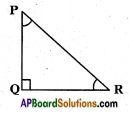In the △PQR
Opposite side = PQ
Hypotenuse = PRQuestion 2.
i) For angle X
ii) For angle Y        (Page No. 271)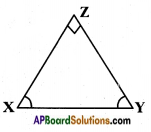In the △XYZ,
i) For angle X
Opposite side = YZ
Hypotenuse = XY
ii) For angle Y
Opposite side = XZ
Hypotenuse = XY

Question 3.
Find (i) sin C (ii) cos C and (iii) tan C in the given triangle.    (Page No. 274)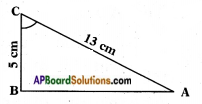By Pythagoras theorem
AC2 = AB2 + BC2
132 = AB2 + 52
AB2 = 169 – 25
AB2 = √144
⇒ AB = √144 = 12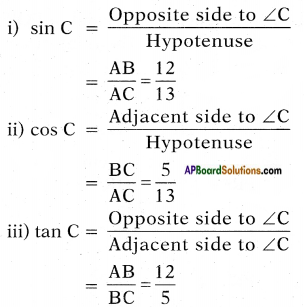Question 4.
In a triangle XYZ, ∠Y is right angle, XZ = 17 cm and YZ = 15 cm, then find (i) sin X (ii) cos Z (iii) tan X.    (Page No. 274)
Given △XYZ, ∠Y is right angle.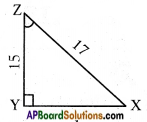By Pythagoras theorem
XZ2 = YZ2 + XY2
172 = 152 + XY2
XY2 = 172 – 152 = 289 – 225
XY2 = 64
XY = √64 = 8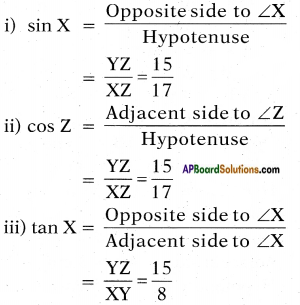Question 5.
In a triangle PQR with right angle at Q, the value of ∠P is x, PQ = 7 cm and QR = 24 cm, then find sin x and cos x.    (Page No. 274)
Given right angled triangle is PQR with right angle at Q. The value of ∠P is x.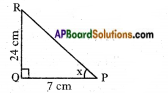By Pythagoras theorem
PR2 = PQ2 + QR2
PR2 = 72 + 242
PR2 = 49 + 576
PR2 = 625
PR2 = √625 = 25
sin x = $$\frac{QR}{PR}$$ = $$\frac{24}{25}$$
cos x = $$\frac{PQ}{PR}$$ = $$\frac{7}{25}$$Try This

Question 1.
Write lengths of “Hypotenuse”, “Opposite side” and “Adjacent side” for the given angles in the given triangles.
1. For angle C
2. For angle A          (Page No. 271)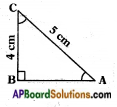By Pythagoras theorem
AC2 = AB2 + BC2
(5)2 = AB2 + 42
25 = AB2 + 16
AB2 = 25 – 16
AB2 = 9
AB = √9 = 3
For angle C:
Opposite side = AB = 3 cm
Adjacent side = BC = 4 cm
Hypotenuse = AC = 5 cm
For angle A:
Opposite side = BC = 4 cm
Adjacent side = AB = 3 cm
Hypotenuse = AC = 5 cm

Question 2.
In a right angle triangle ABC, right angle is at C. BC + CA = 23 cm and BC – CA = 7 cm, then find sin A and tan B.    (Page No. 274)
In a right angle triangle ABC, right angle is at C.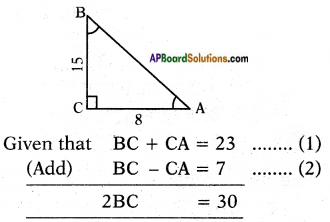BC = $$\frac{30}{2}$$ = 15
BC = 15
Substituting BC = 15 in equation (1)
BC + CA = 23
CA = 23 – BC = 23 – 15
CA = 8
By Pythagoras theorem
AB2 = AC2 + BC2
= 82 + 152
= 64 + 225 = 289
= √289 = 17
sin A = $$\frac{BC}{AB}$$ = $$\frac{15}{17}$$
cos B = $$\frac{AC}{BC}$$ = $$\frac{8}{15}$$

Question 3.
What will be the ratios of sides for sec A and cot A?    (Page No. 275)
sec A = $$\frac{\text { Hypotenuse }}{\text { Adjacent side of the angle } \mathrm{A}}$$
cot A = $$\frac{\text { Adjacent side of the angle } A}{\text { Opposite side of the angle } A}$$Think & Discuss

Question 1.
i) sin x = $$\frac{4}{3}$$ does exist for some value of angle x?
ii) The value of sin A and cos A is always less than 1. Why?
iii) tan A is product of tan and A.        (Page No. 274)
i) The value of sin 0 always lies between 0 and 1. Here sin x = $$\frac{4}{3}$$ which is greater than 1. So, it does not exist.
ii)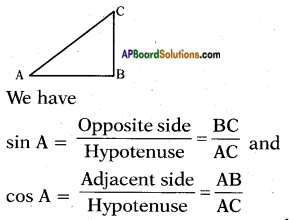We observe in above sin A, cos A, hypotenuse is in denominator which is greater than other two sides
∴ sin A = $$\frac{\text { Opposite side }}{\text { Hypotenuse }}$$ = $$\frac{Nr}{Dr}$$
here denominator is more than numerator. Hence its value will be less than 1.
cos A = $$\frac{\text { Adjacent side }}{\text { Hypotenuse }}$$
here also adjacent side is always less than hypotenuse. Hence its value is also less than or equal to 1.

iii) The symbol tan A is used as an abbreviation for “the tan of the angle A”.
tan A is not the product of “tan” and A. “tan” separated from A’ has no meaning.

Question 2.
Is $$\frac{\sin \mathrm{A}}{\cos \mathrm{A}}$$ equal to tan A?      (Page No. 275)
Yes, $$\frac{\sin \mathrm{A}}{\cos \mathrm{A}}$$ = tan A
Proof: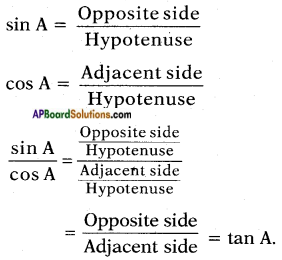Question 3.
Is $$\frac{\cos \mathrm{A}}{\sin \mathrm{A}}$$ equal to cot A?     (Page No. 275)
Yes, $$\frac{\cos \mathrm{A}}{\sin \mathrm{A}}$$ = tan A
Proof: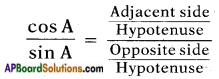cot A = $$\frac{\text { Adjacent side }}{\text { Opposite side }}$$

Do this

Question 1.
Find cosec 60°, sec 60° and cot 60°.       (Page No. 279)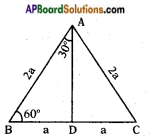Consider an equilateral triangle ABC. Since each angle is 60° in an equilateral triangle, we have ∠A = ∠B = ∠C = 60° and the sides of equilateral triangle is AB = BC = CA = 2a units.
Draw the perpendicular line AD from vertex A to BC as shown in the given figure.
Perpendicular AD acts as “angle bisector of angle A” and “bisector of the side BC” in the equilateral triangle ABC.
Therefore, ∠BAD = ∠CAD = 30°. Since point D divides the side BC into equal halves.
BD = $$\frac{1}{2}$$ BC = $$\frac{2a}{2}$$ = a units.
Consider right angle triangle ABD in the above given figure.
We have AB = 2a and BD = a
Then AD2 = AB2 – BD2
(By Pythagoras theorem)
= (2a)2 – (a)2 = 3a2
From definitions of trigonometric ratios,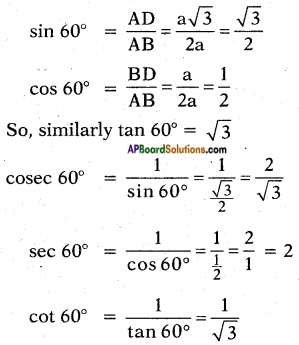Try this

Question 1.
Find sin 30°, cos 30°, tan 30°, cosec 30°, sec 30° and cot 30° by using the ratio concepts.     (Page No. 279)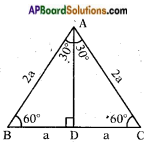Consider an equilateral triangle ABC. Since each angle is 60° in an equilateral triangle, we have ∠A = ∠B = ∠C = 60° and the sides of equilateral triangle is AB = BC = CA = 2a units.
Draw the perpendicular line AD from vertex A to BC as shown in the given figure.
Perpendicular AD acts as “angle bisector of angle A” and “bisector of the side BC” in the equilateral triangle ABC.
Therefore, ∠BAD = ∠CAD = 30°. Since point D divides the side BC into equal halves.
BD = $$\frac{1}{2}$$ BC = $$\frac{2a}{2}$$ = a units.
Consider right angle triangle ABD in the above given figure.
We have AB = 2a and BD = a
Then AD2 = AB2 – BD2
(By Pythagoras theorem)
= (2a)2 – (a)2 = 3a2
Therefore, AD = $$\sqrt{3 a^{2}}$$ = √3a
BD = a, AD = √3a and hypotenuse = AB = 2a and ∠DAB = 30°.
sin 30° = $$\frac{BD}{AB}$$ = $$\frac{a}{2a}$$ = $$\frac{1}{2}$$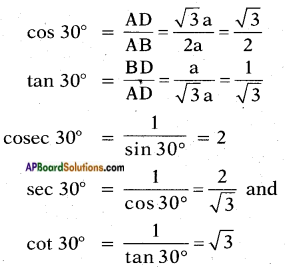Question 2.
Find the values for tan 90°, cosec 90°, sec 90° and cot 90°.     (Page No. 281)
From the adjacent figure, the trigonometric ratios of ∠A, gets larger and larger in △ABC till it becomes 90°.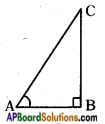As ∠A get larger and larger, ∠C gets smaller and smaller. Therefore, the length of the side AB goes on decreasing. The point A gets closer to point B. Finally when ∠A is very close to 90°, ∠C becomes very close to 0° and the side AC almost coincides with side BC.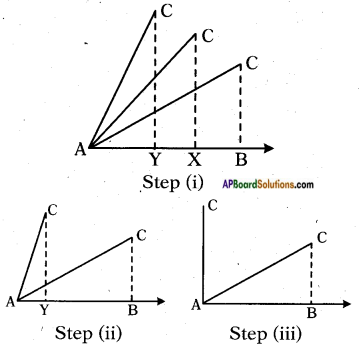∴ AB = 0 and AC = BC = r
From trigonometric ratios
sin A = $$\frac{BC}{AC}$$
sin A = $$\frac{AB}{AC}$$
If A = 90°, then AB = 0 and AC = BC = r,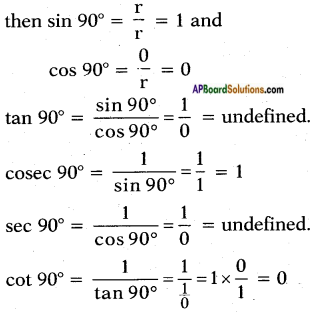Think & Discuss

Question 1.
What can you say about cosec 0° = $$\frac{1}{\sin 0^{\circ}}$$? Is it defined? Why?    (Page No. 280)
sin 0° = 0
cosec 0° = $$\frac{1}{\sin 0^{\circ}}$$ = $$\frac{1}{0}$$ = not defined.
It is not defined.
Reason:
Division by ‘0’ is not allowed, hence $$\frac{1}{0}$$ is indeterminate.

Question 2.
What can you say about cot 0° = $$\frac{1}{\tan 0^{\circ}}$$. Is it defined? Why?    (Page No. 281)
Is it defined? Why?
tan 0° = 0
cot 0° = $$\frac{1}{\tan 0^{\circ}}$$ = $$\frac{1}{0}$$ = undefined.
Reason:
Division by ‘0’ is not allowed, hence $$\frac{1}{0}$$ is indeterminate.

Question 3.
sec 0° = 1. Why?      (Page No. 281)
sec 0° = $$\frac{1}{\cos 0^{\circ}}$$ [∵ cos 0° = 1]
= $$\frac{1}{1}$$ = 1

Question 4.
What can you say about the values of sin A and cos A, as the value of angle A increases from 0° to 90°? (Observe the above table)
i) If A ≥ B, then sin A ≥ sin B. Is it true?
ii) If A ≥ B, then cos A ≥ cos B. Is it true? Discuss.      (Page No. 282)
i) Given statement
“If A ≥ B, then sin A ≥ sin B”
Yes, this statement is true.
Because, it is clear from the table below that the sin A increases as A increases.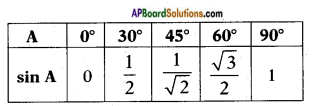ii) Given statement
“If A ≥ B, then cos A ≥ cos B”
No, this statement is not true.
Because, it is clear from the table below that cos A decreases as A increases.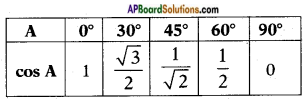Think & Discuss

Question 1.
For which value of acute angle
(i) $$\frac{\cos \theta}{1-\sin \theta}$$ + $$\frac{\cos \theta}{1+\sin \theta}$$ = 4 is true?
For which value of 0° ≤ θ ≤ 90°, above equation is not defined?    (Page No. 285)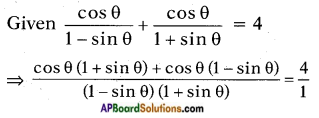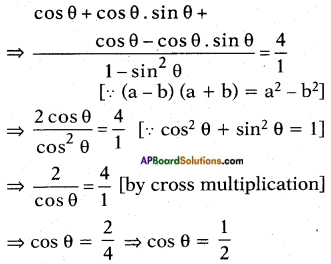⇒ cos θ = cos 60° [from trigonometric ratios table]
⇒ θ = 60°
Given statement is true for the acute angle i.e., θ = 60°.

Question 2.
Check and discuss the above relations in the case of angles between 0° and 90°, whether they hold for these angles or not? So,          (Page No. 286)
i) sin (90° – A) = cos A
ii) cos (90° – A) = sin A
iii) tan (90° – A) = cot A and
iv) cot (90° – A) = tan A
v) sec (90° – A) = cosc A
vi) cosec (90° – A) = sec A
Let A = 30°
i) sin (90° – A) = cos A
⇒ sin (90° – 30°) = cos 30°
⇒ sin 60° = cos 30° = $$\frac{\sqrt{3}}{2}$$

ii) cos (90° – A) = sin A
⇒ cos (90° – 30°) = sin 30°
⇒ cos 60° = sin 30° = $$\frac{1}{2}$$

iii) tan (90° – A) = cot A
⇒ tan (90° – 30°) = cot 30°
⇒ tan 60° = cot 30° = √3

iv) cot (90° – A) = tan A
⇒ cot (90° – 30°) = tan 30°
⇒ cot 60° = tan 30° = $$\frac{1}{\sqrt{3}}$$

v) sec (90° – A) = cosec A
⇒ sec (90° – 30°) = cosec 30°
⇒ sec 60° = cosec 30° = 2

vi) cosec (90° – A) = sec A
⇒ cosec (90° – 30°) = sec 30°
⇒ cosec 60° = sec 30° = $$\frac{2}{\sqrt{3}}$$

So, the above relations hold for all the angles between 0° and 90°.Do this

(Page No. 290)

Question 1.
i) If sin A = $$\frac{15}{17}$$ then find cos A.
Given sin A = $$\frac{15}{17}$$
cos A = $$\sqrt{1-\sin ^{2} A}$$ [From Identity -I]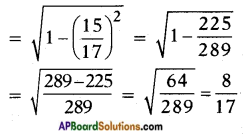ii) If tan x = $$\frac{5}{12}$$, then find sec x. (AS j)
Given tan x = $$\frac{5}{12}$$
We know that sec2 x – tan2 x = 1
sec2 x = 1 + tan2 x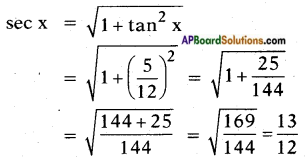iii) If cosec θ = $$\frac{25}{7}$$, then find cot θ.
Given cosec θ = $$\frac{25}{7}$$
We know that cosec2 θ – cot2 θ = 1
cot2 θ = cosec2 θ – 1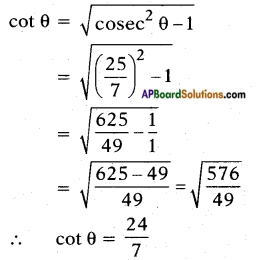Try This

(Page No. 290)

Question 1.

i) $$\frac{\sin ^{2} 15^{\circ}+\sin ^{2} 75^{\circ}}{\cos ^{2} 36^{\circ}+\cos ^{2} 54^{\circ}}$$
Given $$\frac{\sin ^{2} 15^{\circ}+\sin ^{2} 75^{\circ}}{\cos ^{2} 36^{\circ}+\cos ^{2} 54^{\circ}}$$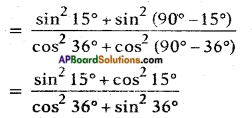[∵ sin (90° – θ) = cos θ; cos (90° – θ) = sin θ]
= $$\frac{1}{1}$$ = 1 [By sin2 θ + cos2 θ = 1]

ii) sin 5° cos 85° + cos 5° sin 85°
Given sin 5° cos 85° + cos 5° sin 85°
= sin 5° . cos (90° – 5°) + cos 5° . sin (90° – 5°)
= sin 5° . sin 5° + cos 5° . cos 5°
[∵ sin (90° – θ) = cos θ; cos (90° – θ) = sin θ]
= sin2 5° + cos2
= 1 [∵ sin2 θ + cos2 θ = 1]

iii) sec 16° cosec 74° – cot 74° tan 16°.
Given sec 16° cosec 74° – cot 74° tan 16°
= sec 16° . cosec (90° – 16°) – cot (90° – 16°) . tan 16°
= sec 16°. sec 16° – tan 16° . tan 16° [∵ cosec (90° – θ) = sec θ; cot (90° – θ) — tan θ]
= sec2 16° – tan2 16°
= 1 [∵ sec2 θ – tan2 θ = 1]Think & Discuss

(Page No. 290)

Question 1.
Are these identities true for 0° ≤ A ≤ 90°? If not for which values of A they are true?
i) sec2 A – tan2 A = 1
Given identity is sec2 A – tan2 A = 1
Let A = 0°
L.H.S. = sec2 0° – tan2
= 1 – 0 = 1 = R.H.S.
Let A = 90°
tan A and sec A are not defined.
So it is true.
∴ For all given values of ‘A’ such that 0° ≤ A ≤ 90° this trigonometric identity is true.

ii) cosec2 A – cot2 A = 1